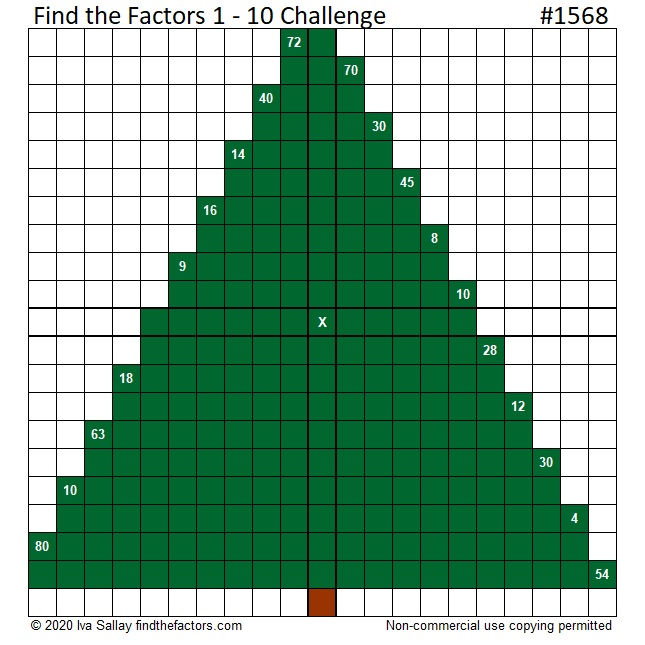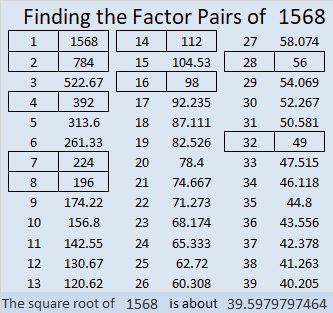# 1568 A Challenging Christmas Tree

### Today’s Puzzle:

Can you write the numbers from 1 to 10 in each of the boldly outlined columns and rows so that each quadrant of this Christmas tree puzzle behaves like a multiplication table? Remember to use logic and not guessing and checking.Here is the same puzzle that won’t use so much ink to print:### 1568 Factor Tree:

Here is one of several possible factor trees for 1568:### Factors of 1568:

• 1568 is a composite number.
• Prime factorization: 1568 = 2 × 2 × 2 × 2 × 2 × 7 × 7, which can be written 1568 = 2⁵ × 7²
• 1568 has at least one exponent greater than 1 in its prime factorization so √1568 can be simplified. Taking the factor pair from the factor pair table below with the largest square number factor, we get √1568 = (√784)(√2) = 28√2
• The exponents in the prime factorization are 5 and 2. Adding one to each exponent and multiplying we get (5 + 1)(2 + 1) = 6 × 3 = 18. Therefore 1568 has exactly 18 factors.
• The factors of 1568 are outlined with their factor pair partners in the graphic below.### More about the Number 1568:

1568 is the difference of two squares SIX different ways:
393² – 391² = 1568,
198² – 194² = 1568,
102² – 94² = 1568,
63² – 49² = 1568,
57² – 41² = 1568, and
42² – 14² = 1568.